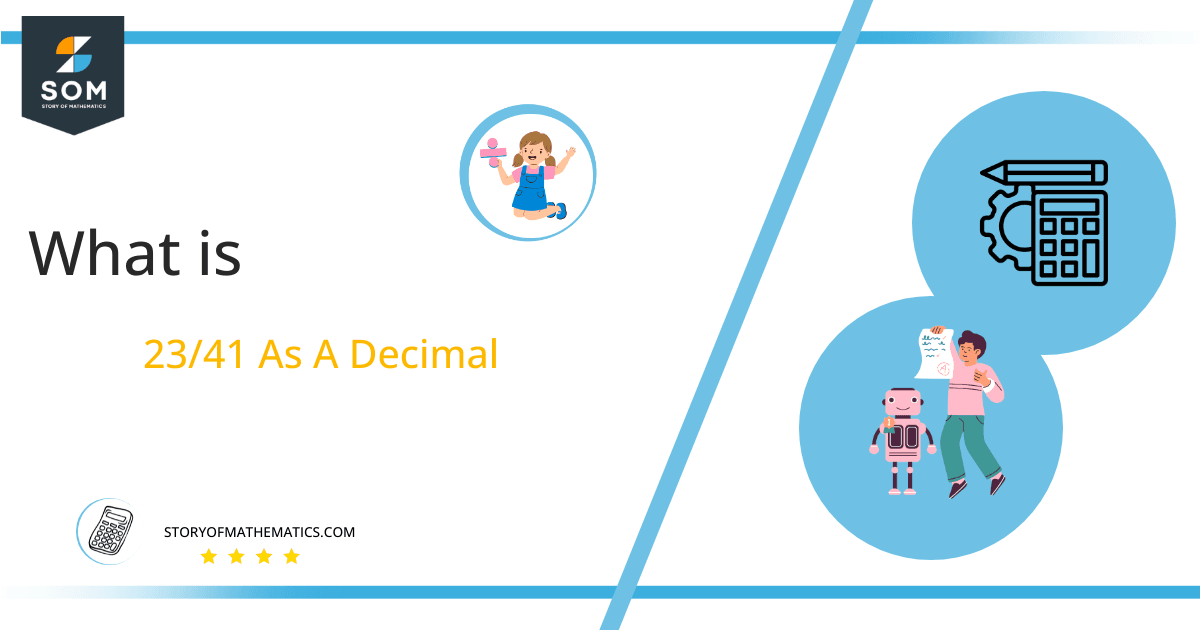# What Is 23/41 as a Decimal + Solution With Free Steps

The fraction 23/41 as a decimal is equal to 0.560.

The long division makes complex problems into simple steps. While performing long division, some steps need to be followed in order and precisely; otherwise, long division can go wrong and other complexes the problem.Here, we are more interested in the division types that result in a Decimal value, as this can be expressed as a Fraction. We see fractions as a way of showing two numbers having the operation of Division between them that result in a value that lies between two Integers.

Now, we introduce the method used to solve said fraction to decimal conversion, called Long Division, which we will discuss in detail moving forward. So, let’s go through the Solution of fraction 23/41.

## Solution

First, we convert the fraction components, i.e., the numerator and the denominator, and transform them into the division constituents, i.e., the Dividend and the Divisor, respectively.

This can be done as follows:

Dividend = 23

Divisor = 41

Now, we introduce the most important quantity in our division process: the Quotient. The value represents the Solution to our division and can be expressed as having the following relationship with the Division constituents:

Quotient = Dividend $\div$ Divisor = 23 $\div$ 41

This is when we go through the Long Division solution to our problem.Figure 1

## 23/41 Long Division Method

We start solving a problem using the Long Division Method by first taking apart the division’s components and comparing them. As we have 23 and 41, we can see how 23 is Smaller than 41, and to solve this division, we require that 23 be Bigger than 41.

This is done by multiplying the dividend by 10 and checking whether it is bigger than the divisor or not. If so, we calculate the Multiple of the divisor closest to the dividend and subtract it from the Dividend. This produces the Remainder, which we then use as the dividend later.

Now, we begin solving for our dividend 23, which after getting multiplied by 10 becomes 230.

We take this 230 and divide it by 52; this can be done as follows:

230 $\div$ 41 $\approx$ 5

Where:

41 x 5 = 205

This will lead to the generation of a Remainder equal to 230 – 205 = 25. Now this means we have to repeat the process by Converting the 25 into 250 and solving for that:

250 $\div$ 41 $\approx$ 6

Where:

41 x 6 = 246

This, therefore, produces another Remainder which is equal to 250 – 246 = 4.

Finally, we have a Quotient generated after combining the three pieces of it as 0.560=z, with a Remainder equal to 4.Images/mathematical drawings are created with GeoGebra.